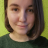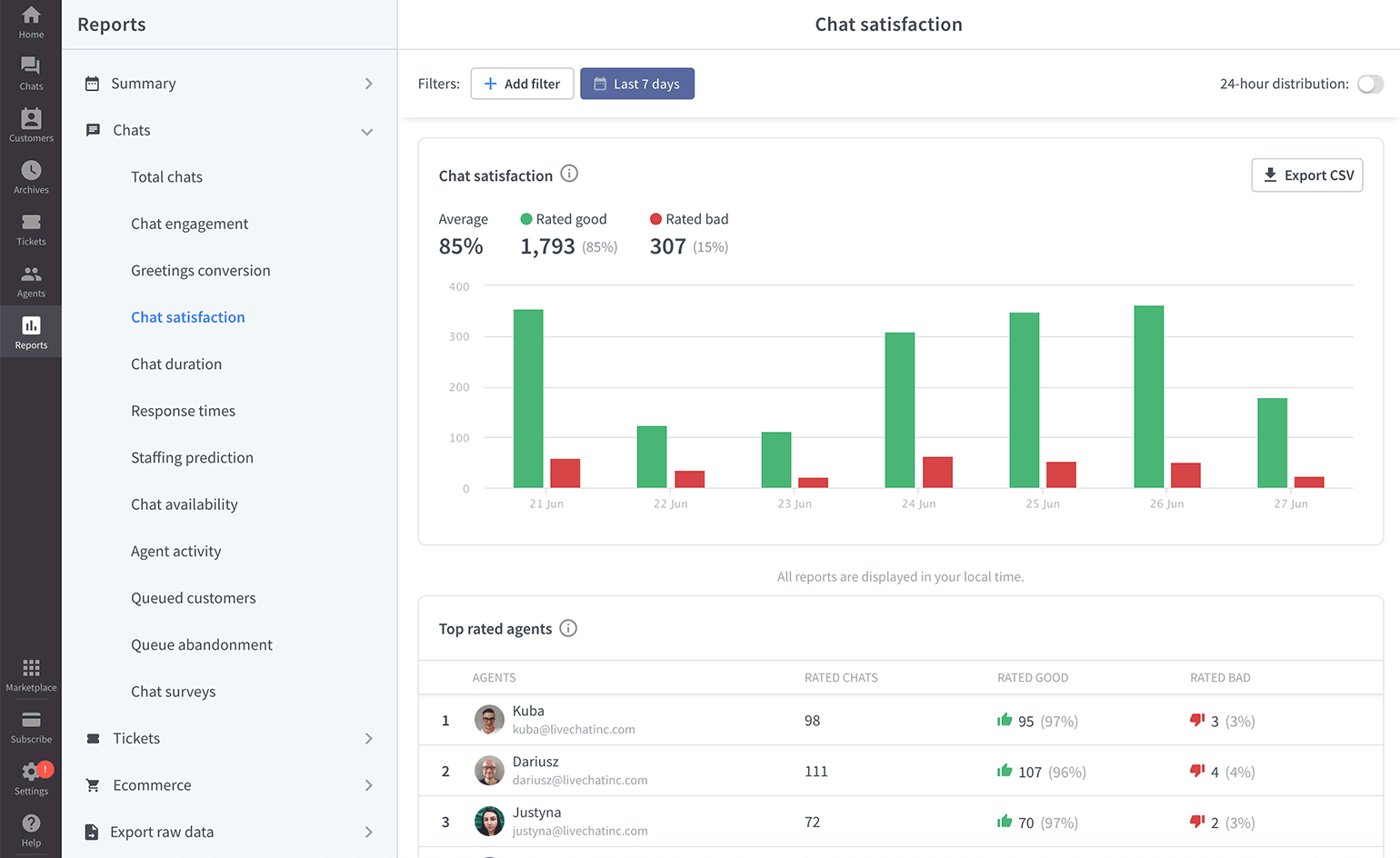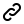# How we calculate agent rankingsAlicja Pawliczak2 min readupdated: Sep 20, 2022

As you might have noticed we don’t use a simple average rating formula (so avg=positive ratings / total ratings) to rank agents in the chat and ticket satisfaction reports.Instead, we use Wilson score, which lets us calculate the probability of getting a positive rating, taking into account both the number of positive and negative ratings in relation to all ratings.

## Why we use Wilson scoreStill not sure why we chose some complicated formula instead of the average?

Let’s look at an example:

Jake has 2 positive ratings and 0 negative ratings, while Anna has 10 positive ratings and 2 negative ratings. Who do you think should be ranked first? I bet you said Anna.

If we used the average to calculate rankings, it would be Jake that is ranked higher than Anna. Because 100% of his ratings are positive in comparison to Anna’s 83%.

That’s why we use Wilson score (you can see the exact formula for calculating it below). It calculates the probability of getting a positive rating: which is 52% for Anna and 33% for Jake. Meaning that Anna is ranked higher than Jake. Which makes things fair.

## Calculate Wilson score for your agentsHave some spare time on your hands? Check if Wilson score actually works. Here is the formula we use to calculate it:

Constants:
Z=2.69 (confidence level)

Pre-calculated values:
n=number of positive ratings + number of negative ratings (total number of ratings)
p=number of positive ratings / n (probability of a positive rating)
zznf=Z * Z/(4 * n) (constant for keeping the formula short)

Formula:
Wilson score = (p + 2 * zzfn - Z * √ ((zzfn / n + p * (1 - p)) / n)) / (1 + 4 * zzfn)

And an example:
Jake: 2 positive, 0 negative
Anna: 10 positive, 2 negative

Wilson score for each agent using the formula provided above:
Anna: 0.5194200469 (52%)
Jake: 0.3312764381 (33%)

The higher the score, the higher the probability of getting a positive rating. Which translates to a higher ranking of the agent.

Don’t have time to do the maths by yourself? Not a problem. We prepared a Google Sheets document, that you can copy and fill in with your agents’ ratings. The score will be calculated automatically.### Got it!### Thank you!

We’re happy to help.

### RELATED ARTICLES

#### Discrepancies in LiveChat reports

Find out what are the possible discrepancies in LiveChat reports and what do they mean! Because not all discrepancies are bad.

#### Reports section overview

LiveChat offers a wide range of chat and tickets reports. Use them to fine-tune your implementation and spot areas that can be optimized and more efficient.